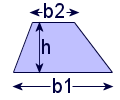# Calculate the Area of Common Shapes

Square: $$Area = (Side Length)^2$$

The area of a square is the length of a side, squared.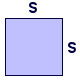Rectangle: $$Area = (Length)*(Width)$$

The area of a rectangle is the length multiplied by the width.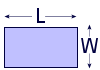Circle: $$Area = \pi*r^2$$

The area of a circle is pi (about 3.14) times the radius squared.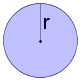Triangle: $$Area = \frac{1}{2}*(Base)*(Height)$$

The area of a triangle is one half the base times the height.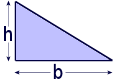Trapezoid: $$Area = \frac{1}{2}*(B_1+B_2)*(Height)$$

The area of a trapezoid is one half the sum of the bases times the height.